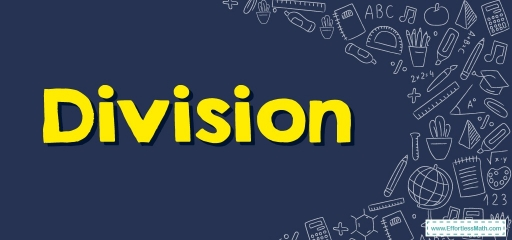# DivisionThe division is the process of putting a group of objects into equal parts. It’s an example of the $$4$$ fundamental math operations, which give a fair outcome of sharing.

The division is the procedure of repetitive subtracting. It’s the opposite of multiplication. Whenever we know a fact of multiplication, it’s possible to get a division fact.

The primary objective division is to find the number of equal groups or the number in each group whenever you share fairly.

Whilst doing number division, you break down bigger numbers to lesser numerals such as multiplying these lower so they’ll be equivalent to the higher number taken.

## Division Symbol

The division is represented by a numerical symbol consisting of a small horizontal line that has a dot above and below the line. There are $$2$$ standard division symbols which denote the division of $$2$$ numbers. These are $$÷$$ and $$/$$.

## Unique names for each Division character

Each of the parts concerned in a division equation has a unique name.

Dividend $$÷$$ divisor $$=$$ quotient

Dividend: Dividend is the figure being divided in the division procedure.

Divisor: Numeral that the dividend is being divided by is known as the divisor.

Quotient: Quotient is the answer gotten during a division procedure.

Reminder: The value remaining or that’s left out which isn’t part of a group

## Unique facts concerning division

• Whenever you divide a number by $$1$$, the result is always going to be your original digit.
• Division by zero is undefined.
• Division of the exact same dividend and divisor is constantly $$1$$.

### Division – Example 1:

Find the missing number.

$$115 \ \div$$ ____ $$=5$$

Solution:

The inverse operation of multiplication is division. Because $$5$$ multiplied by $$23$$ equals $$115$$, then $$115$$ divided by $$5$$ equals $$23$$.

### Division – Example 2:

Lisa wants to buy $$32$$ candles for a party. If there are $$4$$ candles in each pack, how many packs of candles should she buy?

Solution:

In this question, the candles are in packs of $$4$$. So by dividing $$32$$ by $$4$$, we can find the number of packs that Lisa needs to buy. So, $$32 \ \div \ 4=8$$

## Exercises for Division

Solve

1. $$\color{blue}{64 ÷ 8}$$
2. $$\color{blue}{72 ÷ 18 }$$
3. $$\color{blue}{99 ÷ 11 }$$
4. $$\color{blue}{ 124 ÷ 4 }$$
1. $$\color{blue}{8}$$
2. $$\color{blue}{ 4}$$
3. $$\color{blue}{9}$$
4. $$\color{blue}{31}$$

### What people say about "Division - Effortless Math: We Help Students Learn to LOVE Mathematics"?

No one replied yet.

X
30% OFF

Limited time only!

Save Over 30%

SAVE $5 It was$16.99 now it is \$11.99